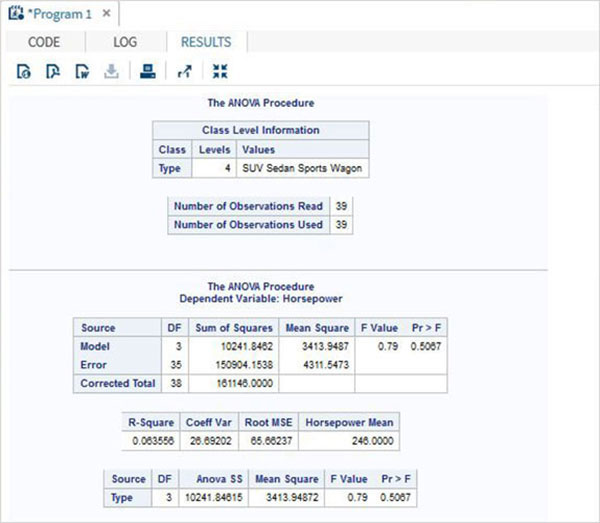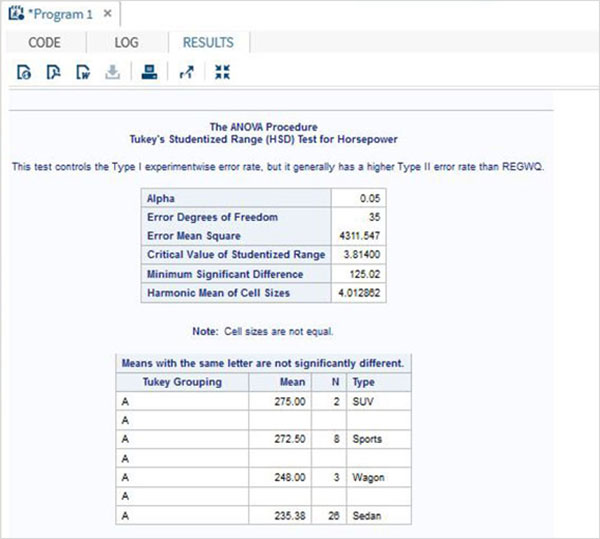# SAS - One Way Anova

ANOVA stands for Analysis of Variance. In SAS it is done using PROC ANOVA. It performs analysis of data from a wide variety of experimental designs. In this process, a continuous response variable, known as a dependent variable, is measured under experimental conditions identified by classification variables, known as independent variables. The variation in the response is assumed to be due to effects in the classification, with random error accounting for the remaining variation.

### Syntax

The basic syntax for applying PROC ANOVA in SAS is −

```PROC ANOVA dataset ;
CLASS Variable;
MODEL Variable1 = variable2 ;
MEANS ;
```

Following is the description of the parameters used −

• dataset is the name of the dataset.

• CLASS gives the variables the variable used as classification variable.

• MODEL defines the model to be fit using certain variables from the dataset.

• Variable_1 and Variable_2 are the variable names of the dataset used in analysis.

• MEANS defines the type of computation and comparison of means.

## Applying ANOVA

Let us now understand the concept of applying ANOVA in SAS.

### Example

Lets consider the dataset SASHELP.CARS. Here we study the dependence between the variables car type and their horsepower. As the car type is a variable with categorical values, we take it as class variable and use both these variables in the MODEL.

```PROC ANOVA DATA = SASHELPS.CARS;
CLASS type;
MODEL horsepower = type;
RUN;
```

When the above code is executed, we get the following result −## Applying ANOVA with MEANS

Let us now understand the concept of applying ANOVA with MEANS in SAS.

### Example

We can also extend the model by applying the MEANS statement in which we use Turkey's Studentized method to compare the mean values of various car types.The category of car types are listed with the mean value of horsepower in each category along with some additional values like error mean square etc.

```PROC ANOVA DATA = SASHELPS.CARS;
CLASS type;
MODEL horsepower = type;
MEANS type / tukey lines;
RUN;
```

When the above code is executed, we get the following result −